# math-for-computer-scienceImportant Math Topics for Data Structures and Algorithms

Just like programming, math is one of the core parts of learning data structures and algorithms. We mainly use math to analyse efficiency of various algorithms. But sometimes, the problem itself contains mathematical property or requires some mathematical insight to find a solution.Find prime numbers in a range: Sieve of Eratosthenes

The sieve of Eratosthenes is an ancient and efficient algorithm for finding all primes numbers from 2 to n. This algorithm finds all the prime numbers in a segment using O(nlog⁡log⁡n) operations.Check if a number is prime or not: Primality Test

Given a positive integer n, write a program to check if the number is prime or not. A number n > 1 is said to be a prime number if 1 and n are its only factors. In other words, a prime number is a number that is divisible only by two numbers itself and one.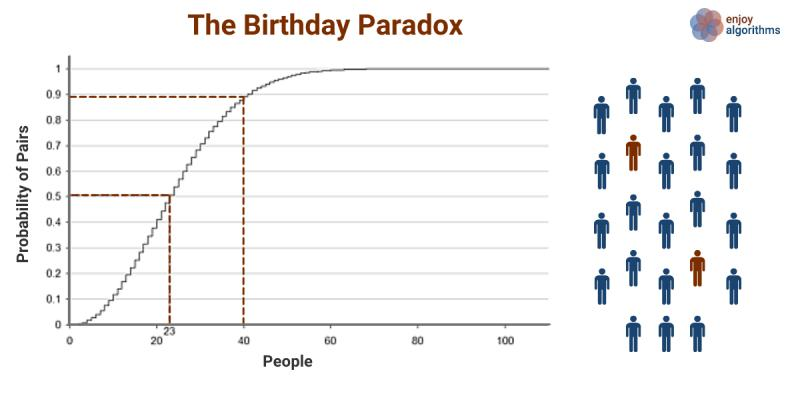The birthday paradox is strange and counter-intuitive. It's a "paradox" because our brain find it difficult to handle the compounding power of exponents. Real-world applications for this include a cryptographic attack called the "birthday attack".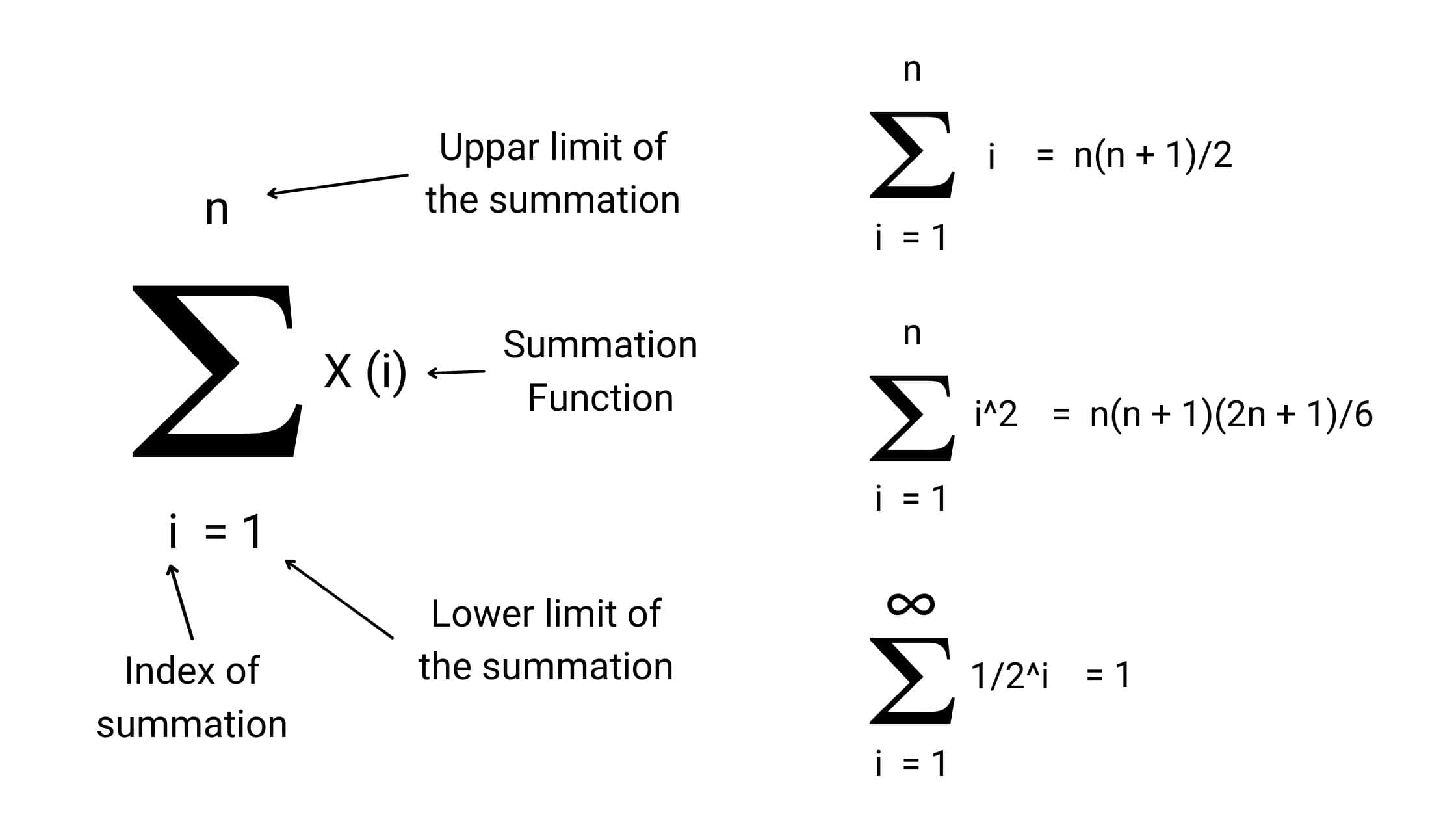Visual Proof: The Sum of Important Mathematical Series

The summation formulas are used to calculate the sum of the sequence. In this blog, we have discussed the visual proofs: the sum of numbers from 1 to n (arithmetic series), the sum of infinite geometric series, the sum of squares of numbers from 1 to n, etc.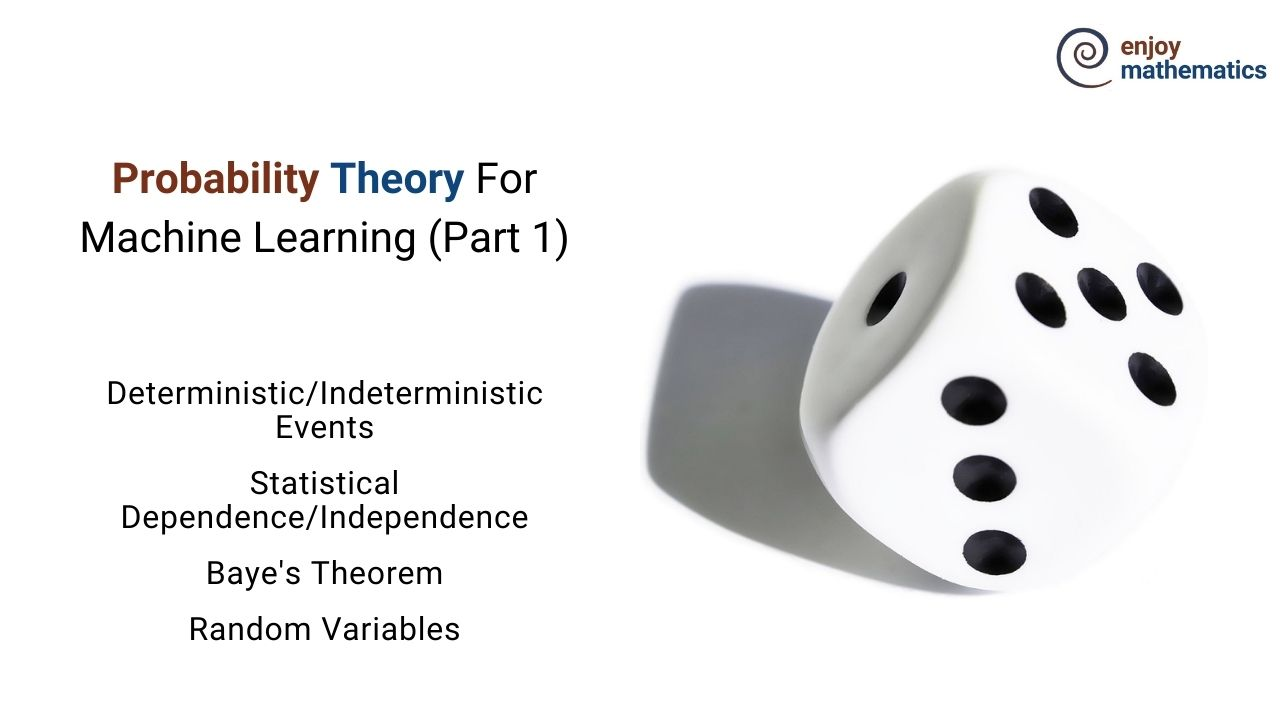Probability Theory For Machine Learning (Part 1)

Originated from the “Games of Chance,” probability in itself is a branch of mathematics concerned about how likely it is that a proposition is true.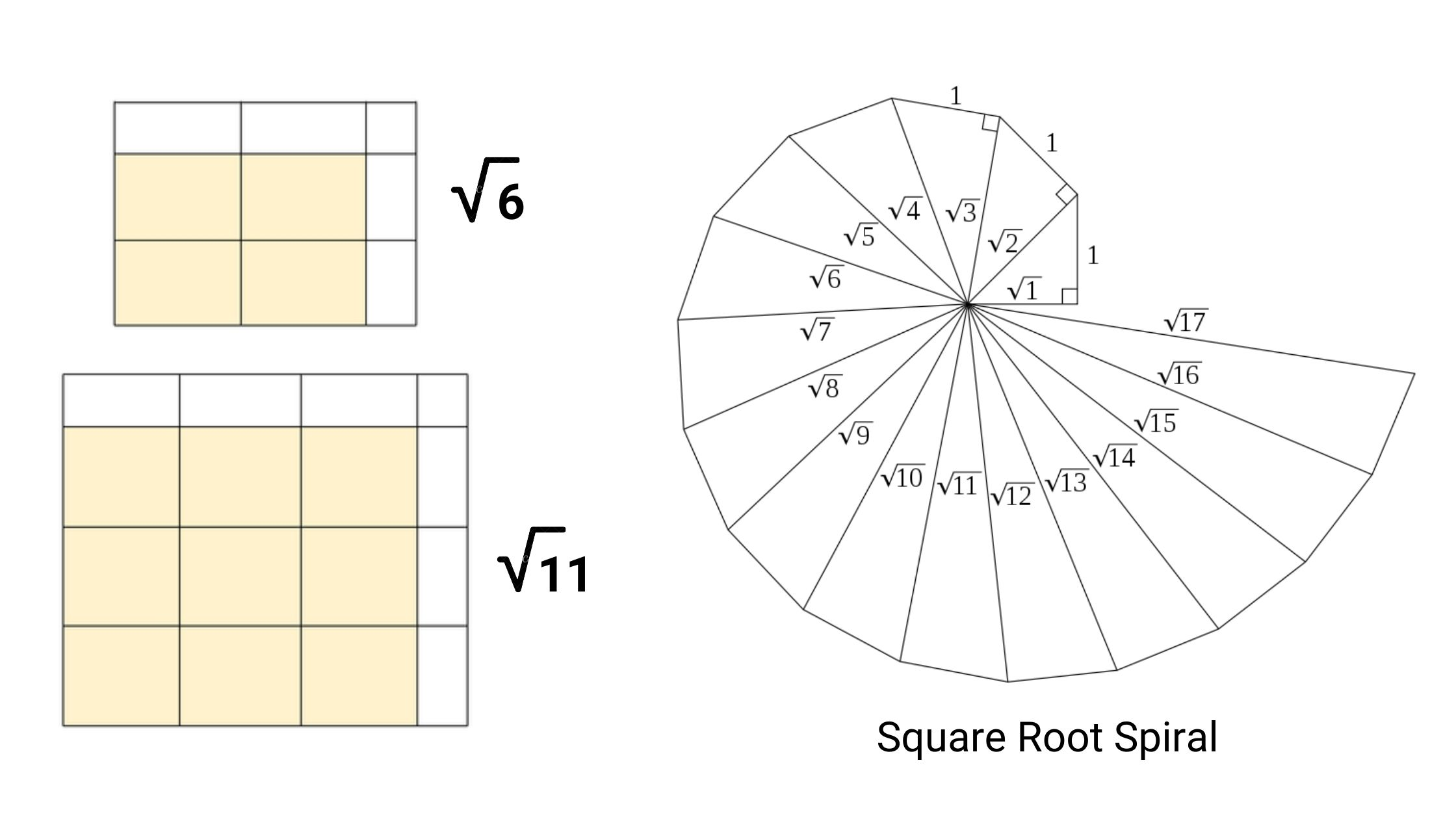Find Square Root of a Number

Given a natural number n, find the largest integer less than or equal to √n. This can be seen as a search problem where the search space S is the set {1, . . . , n}, and the number desired is the floor(√n), i.e., the largest integer that is less than or equal to √n.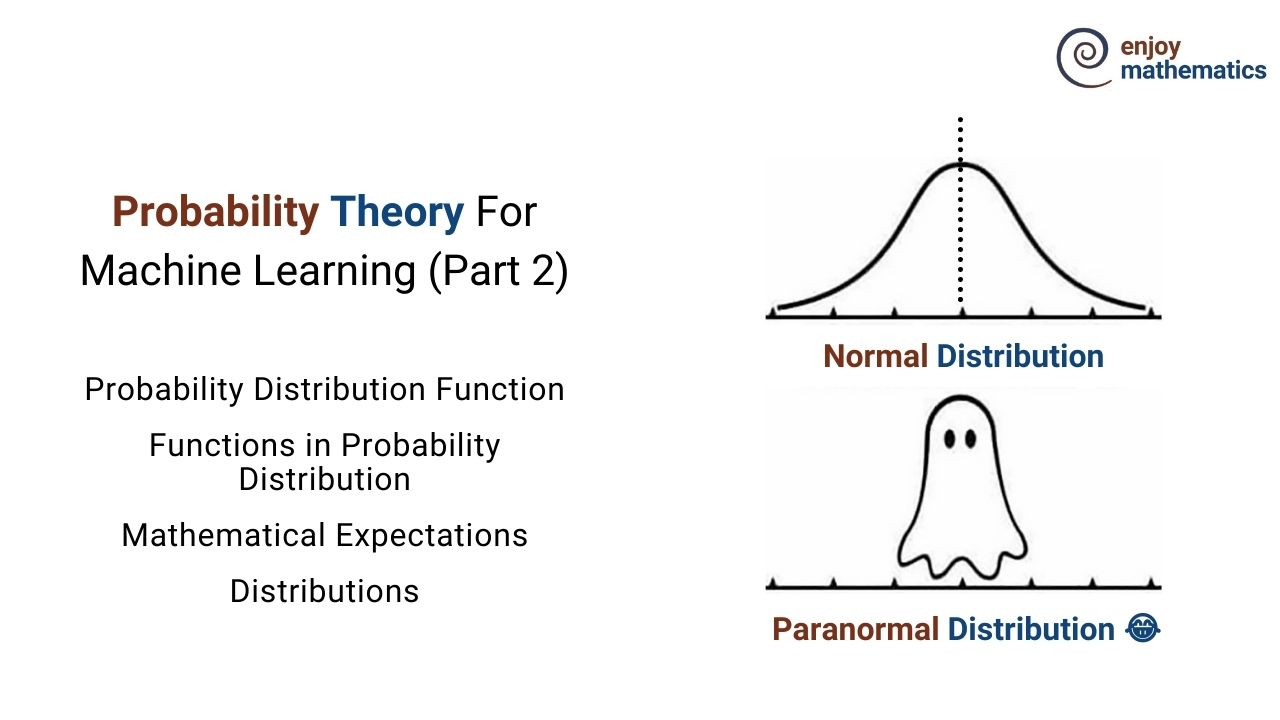Probability Distribution Function For Machine Learning

The Probability distribution lists the probabilities of the events that happened in any given random experiment. For example rolling a dice.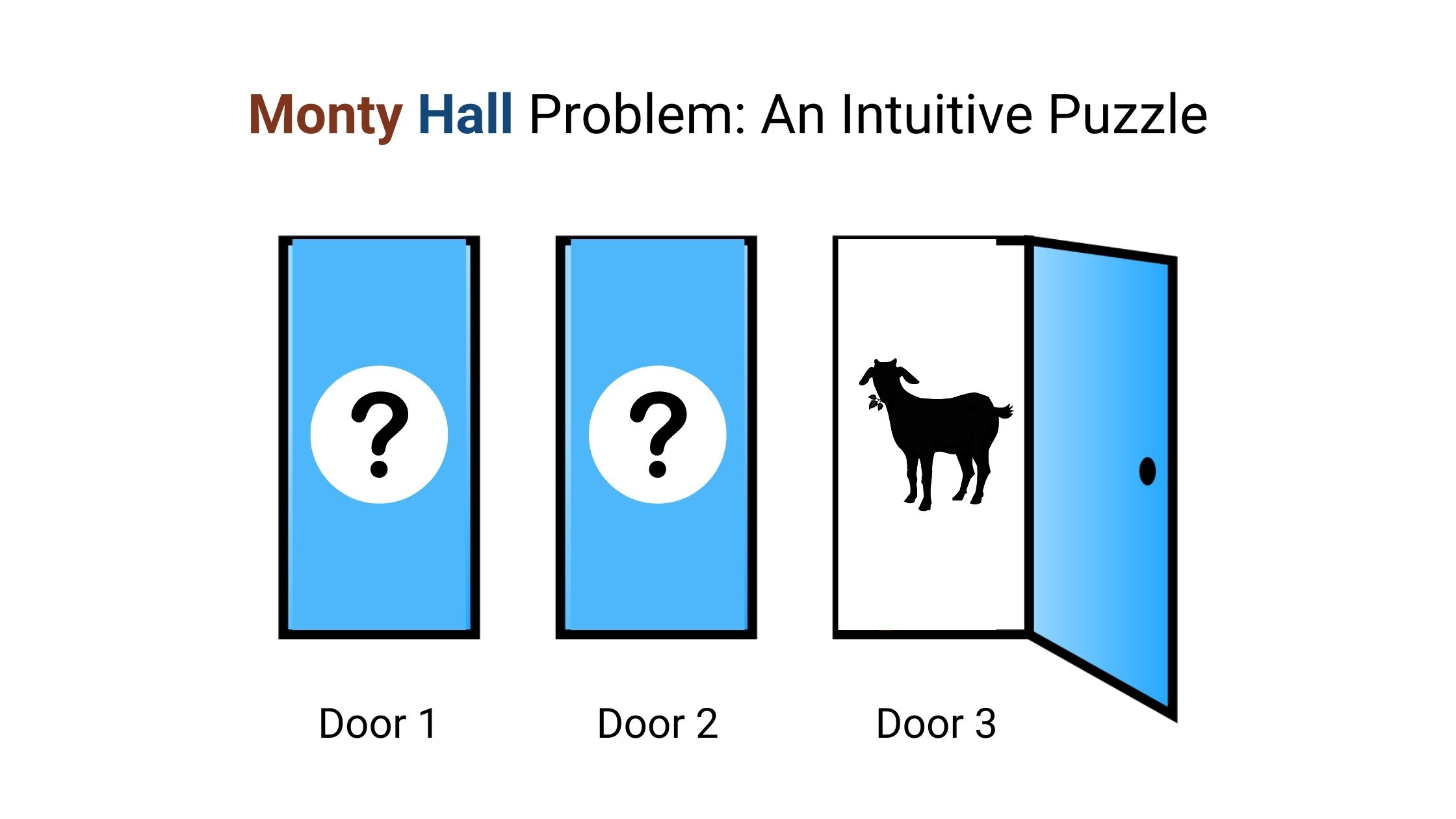Monty Hall Problem

There are 3 doors behind which are two goats and a car. You pick door 1 hoping for the car but don’t open it right away. Monty Hall, the game show host who knows what's behind the doors, opens door 3, which has a goat. Here's the game: do you want to pick door No. 2? Is it to your advantage to switch your choice?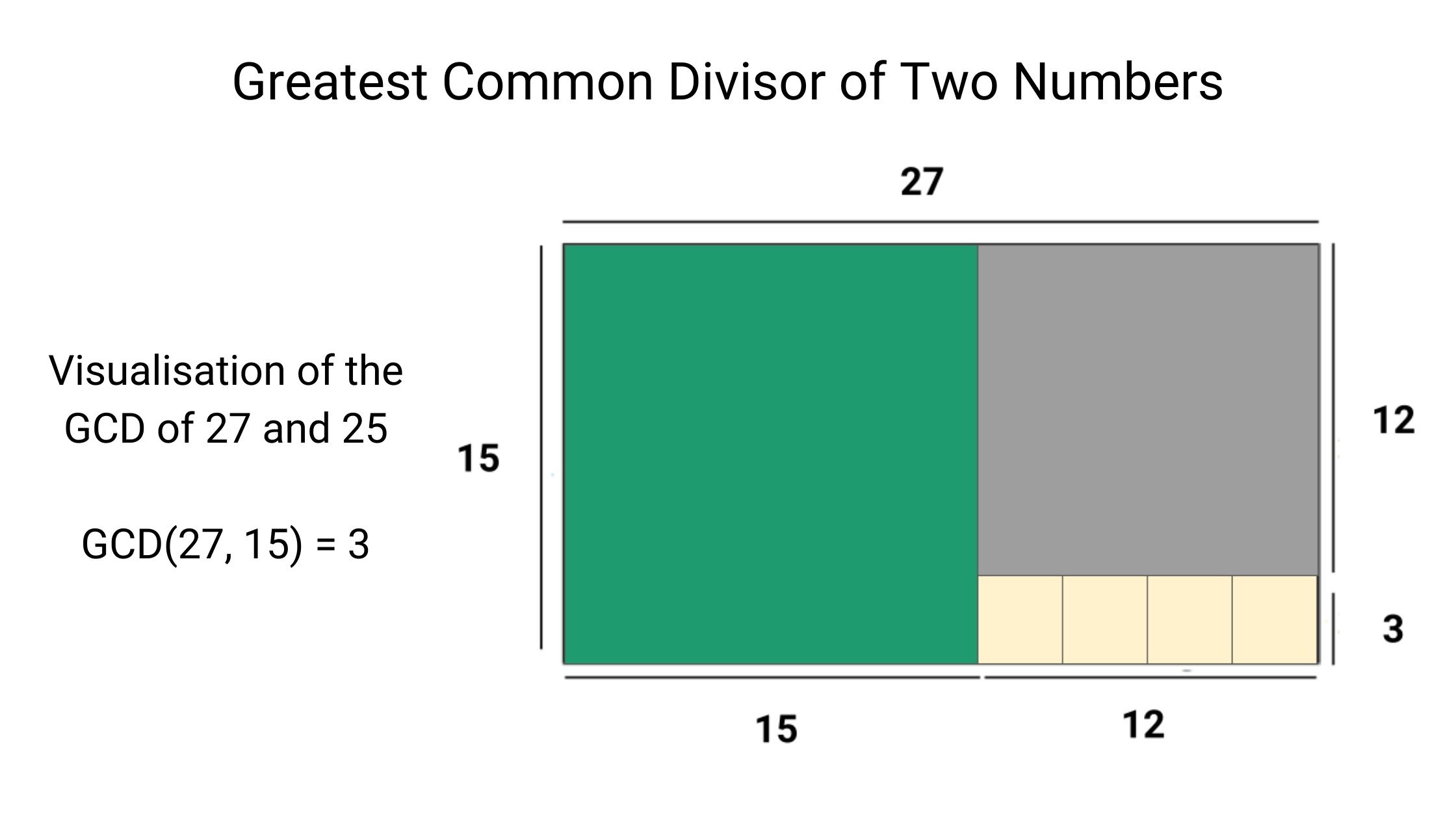Find GCD of Two Numbers: Euclidean Algorithm

Given two non-negative integers, m and n, we have to find their greatest common divisor or HCF. It is the largest number, a divisor of both m and n. The Euclidean algorithm is one of the oldest and most widely known methods for computing the GCD of two integers.

© 2020 Code Algorithms Pvt. Ltd.